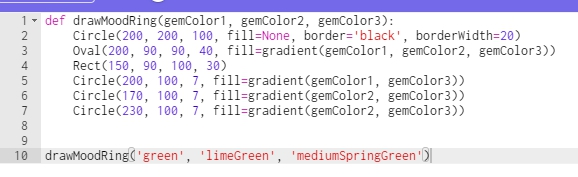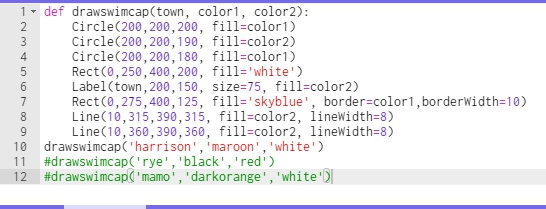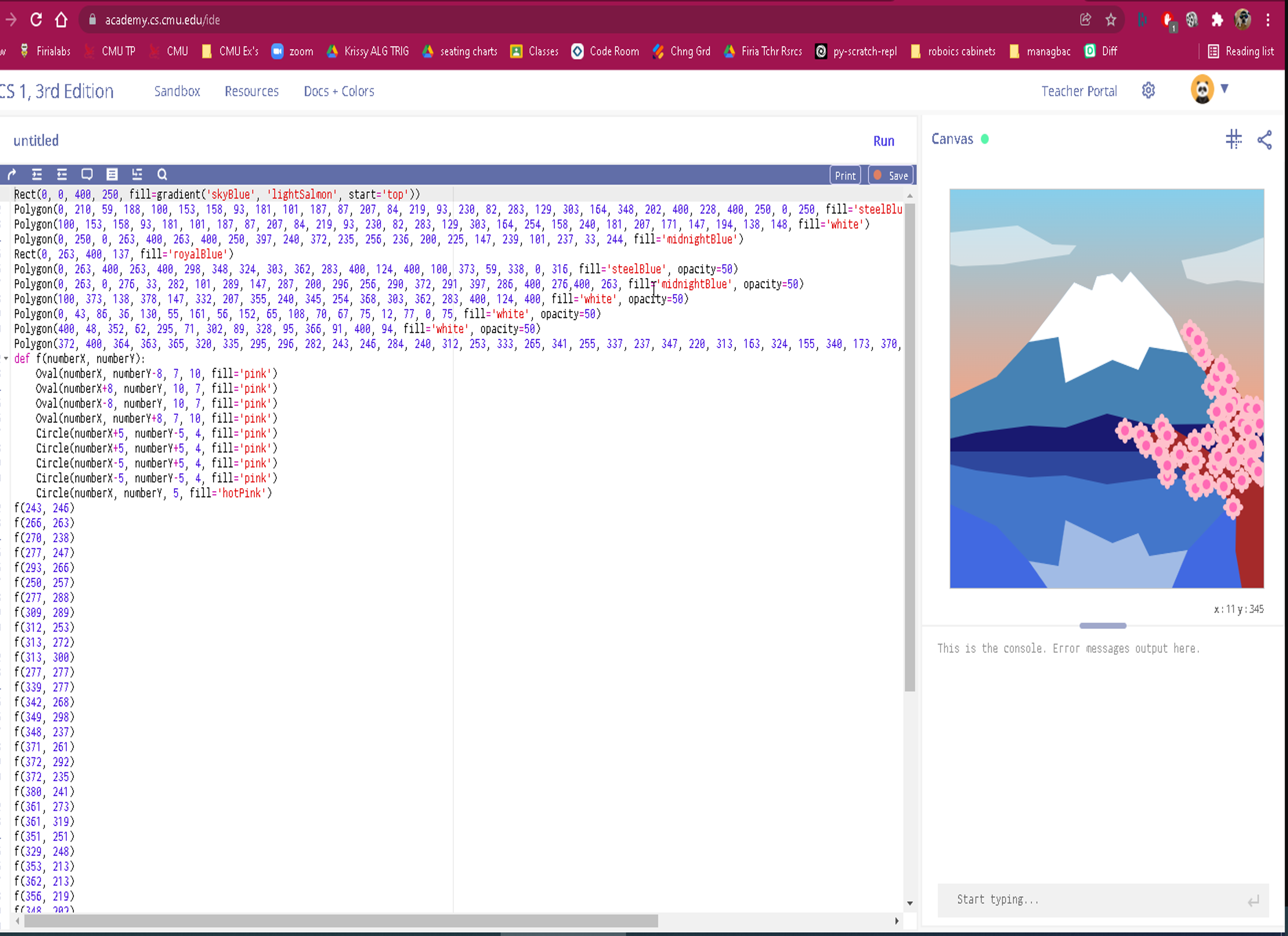# Unit 2 Extra Credit

In addition to the required Unit 2 Creative Task, if you want some extra credit, you can do the following assignment:

Research how groups work in CMU ( see the docs)

Write a program that

• includes two functions
• minimum of 5 objects
• A group comprised of at least 3 objects
• One of the functions must change the properties of all things in the group.

You can write this in one of empty creative a tasks.

# CMU Unit 2, creative task – mousepress mousedown

• minimum of 2 shapes
• minimum of 2 functions
• 1 must be a mouse function (mousePress, mouseMove, mouseRelease)
• the other function can be any function at all
• one of the functions must change a property (fill, width etc) of the shape

After you are done this, maybe you want to try this.

# 2-d Arrays OOP Project

Let’s write a program for something like PowerSchool that helps organize a seating chart for students.  There is a tester file at the page’s bottom.

First class : Student  , from https://mrmonline.org/student-class/

Class SeatingChart

This class mainly manages a 2-d  array of student objects. Note: an empty seat in the chart will be designated by a null value.

### Constructor(s)

• public SeatingChart(int period, String teacher, String _rosterName) Initializes parameters to respective arguments and creates a 2-d array  of null objects with default dimensions DEFAULT_ROWS , DEFAULT_COLS
• public SeatingChart(int period, String teacher, String _rosterName, Student[][] table)

### Methods:

public int countEmptySeats() ; //returns the number of empty seats. As noted earlier, an empty seat is represented by a null value in the 2-d array of student s

Student[][] getStudentChart()  @returns chart

public int[] indexOf(Student stu);  returns a 2 element array representing the row and column position of stu in chart ; [-1][-1] should be returned if stu is not in chart

public boolean setSeat(int row, int col, Student stu) -  places Student Stu  at that row and column .  This can only be done if there is no student in that location. If a student already inhabits that row and column then the method should return false and not make any changes to chart .

public void swap(Student s1, Student s2) // this swaps the locations of s1 and s2

public Student getStudent(int row, int col) returns the student at the given row and col ; or null if the seat is empty

public void removeStudentAt(int row, int col)   sets value of object at  row  and  col  to  null

public boolean equals(SeatingChart other) // returns true if all aspects of  other are equivalent to self.

public String toString(); //follow the conventions we have been using

public Student oldest() // returns the student object with the greatest age

Extra credit

public void randomize() // this randomizes the chart. This extra credit method is only available if you first meet with me to explain what I expect out of this.

SeatingChartTesterv2.java :

``````import java.util.Arrays;

public class SeatingChartTesterv2
{
public SeatingChartTesterv2()
{
Student amelia = new Student("Amelia", 17);
Student antonioooo = new Student("antonioooo", 14);

SeatingChart period3 = new SeatingChart(3, "Mr. M", "A Days");

int correct = 0;
int wrong  = 0;

Student[][] currentChart = period3.getStudentChart();
if(currentChart.length == SeatingChart.DEFAULT_COLS && currentChart.length == SeatingChart.DEFAULT_ROWS)
correct++;
else
{
p("Default constructor's 2-d Array incorrect dimensions");
wrong++;
}

boolean result = period3.setSeat(5,6, amelia );

if(result)
correct++;
else
{
p("setSeat() return value error");
}

Student[][] chartNow = period3.getStudentChart();
if(chartNow == amelia)
{
correct++;

// Student getStudent(int row, int col)
if( period3.getStudent(5,6) == amelia)
correct++;
else
{
p("Error, looks like getStudent() ");
}

}
else
{
p("A) erorr, probably from setSeat() or getStudentChart() ");
wrong++;
}

int[] locaxn = period3.indexOf(amelia);
if(locaxn==5 && locaxn == 6)
correct++;
else
{
p("B) setSeat() or indexOf() error");
wrong++;
}
result =period3.setSeat(5,6, antonioooo );

if(result== false)
correct++;
else
{
p("C) setSeat() return value error");
wrong++;
}

String st = period3.toString();

if(st.indexOf("SeatingChart") == 0)
correct++;
else{
p("toString() error. ClassName should be first characters");
wrong++;
}

if(st.contains("Mr. M"))
correct++;
else{
p("toString() error. ");
wrong++;
}

if(st.contains("A Days"))
correct++;
else{
p("toString() error. ");
wrong++;
}
//new seating chart
Student tim = new Student("tim");
Student[][]  someKids= {
{ new Student(), tim , null},
{ new Student(), new Student("joe", 12), null, null,null},
{ new Student(), new Student("alfjdj", 999), null},
{ new Student(), new Student("sss", 5), null},
};

SeatingChart period4 = new  SeatingChart(4, "MR M", "p4", someKids);

//make sure that someKids was actually set to the chart

Student[][] p4Chart = period4.getStudentChart();
if( Arrays.equals(p4Chart , someKids))
correct++;
else
{
wrong++;
p("Error . Looks like you did not correctly instantiate 2-d array in constructor ");
}

boolean isCorrect = true;
Student oldest  = null ;
try{
oldest = period4.oldest();
}
catch(NullPointerException e){
isCorrect = false;
p("Error @ oldest(), you cannot call .getAge() on a null object");
}

if(isCorrect && oldest != null && oldest.getAge() ==999)
correct++;
else
{
wrong++;
p("Error @ oldest()");
}
locaxn = period4.indexOf(tim);

System.out.print(locaxn);
System.out.print(locaxn);

if(locaxn==0 && locaxn == 1)
correct++;
else
{
p("A incorrect student. Could be constructor");
wrong++;
}

if ( period4.getStudent(0,1) == tim )
correct++;
else
{
p("B incorrect student. Could be constructor, getStudent()");
wrong++;
}
///

int emptySeats = period4.countEmptySeats();

if(emptySeats == 6)
correct++;
else
{
p("countEmptySeats() error");
wrong++;
}

Student vinnie = new Student("Vinnie", 16);
Student yuya = new Student("Yuya", 18);
Student[][]  p5Kids = {
{ yuya, vinnie}
};

SeatingChart period5 = new  SeatingChart(4, "MR M", "p4", p5Kids);

int[]  yuyaLocation_befo = period5.indexOf(yuya);
int[]  vinniaLocation_befor = period5.indexOf(vinnie);

period5.swap(vinnie,yuya) ;
int[]  yuyaLocation_now = period5.indexOf(yuya);
int[]  vinniaLocation_now = period5.indexOf(vinnie);

if( yuyaLocation_now == vinniaLocation_befor &&  yuyaLocation_now == vinniaLocation_befor  )
correct++;
else
{
p("Error Swap");
wrong++;
}
if( vinniaLocation_now == yuyaLocation_befo &&  vinniaLocation_now == yuyaLocation_befo  )
correct++;
else
{
p("Error Swap");
wrong++;
}
////
//

Student rany = new Student("rany", 16);
Student[][]  p6Kids = {
{ rany, null,null,null},
{ null, null,null,null}
};

SeatingChart period6 = new  SeatingChart(4, "MR M", "p4", p6Kids );
period6.removeStudentAt(0,0);
if( period6.getStudent(0,0) == null )
correct++;
else
{
p("Error @ removeStudentAt() ");
wrong++;
}

if( period5.equals(period6) == false)
correct++;
else
{
p("A Error @ equals() ");
wrong++;
}
if( period5.equals(period5) )
correct++;
else
{
p("B Error @ equals() ");
wrong++;
}

SeatingChart period6Clone = new  SeatingChart(4, "MR M", "p4", p6Kids );

if( period6.equals(period6Clone) )
correct++;
else
{
p("C Error @ equals() ");
wrong++;
}

p("----------------------------------");
p("Correct : " + correct);
p("Wrong : " + wrong);

}//end of constructor

void p(String x) { System.out.println(x); }
void p(int x) { System.out.println(x); }
void p(boolean x) { System.out.println(x); }
void p(double x) { System.out.println(x); }
void p2d(int[] x) { System.out.println(x + ",  " + x); }

}``````
SeatingChartTester

# CMU Creative Task Function and Parameter

Use a function and a parameter to draw a picture

You must

• use a function
• the function must include at least 1 parameter
• call the function
• the function must draw a shape